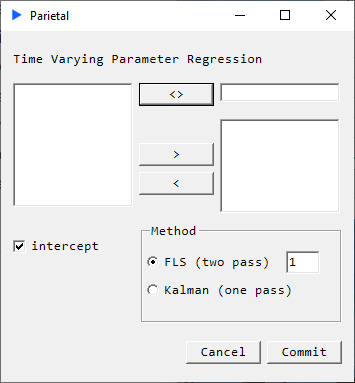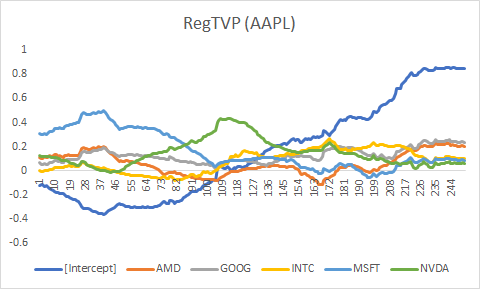# RegTVP

## Time Varying Parameter Regression#### Description#

When analysing time series, it is often the case that state dynamics change over time and often radically so. In such cases analysts often use windowed regressions however it is usually unclear what the appropriate windowing parameter ought to be. There are ways around this. Here we implement two such methods using Flexible Least Squares and Kalman Filtering.

FLS seeks to minimize the following relation: $$C(\beta, \mu) = \sum_{t=1}^{T}(y_{t}-x_{t}^{'}\beta_{t})^2 + \mu \sum_{t=1}^{T-1}\zeta_{t}$$ where $\zeta_{t} = (\beta_{t+1} - \beta_{t})^{'}(\beta_{t+1} - \beta_{t})$ and $\mu$ is a user defined parameter.

The higher $\mu$ is the smoother the estimated parameters and closer they resemble OLS. The closer $\mu$ is to 0, the more ‘jittery’ and responsive the parameters will be.

“The FLS solution is defined to be the collection of all coefficient sequence estimates $\beta$ which yield vector-minimal sums of squared residual measurement and dynamic errors for the given observations–i.e, which attain the “residual efficiency frontier”. The frontier characterizes the efficient attainable trade-offs between residual measurement error and residual dynamic error. In particular; the frontier reveals the cost in terms of residual measurement error that must be paid in order to attain the zero residual dynamic error (time-constant coefficients) required by ordinary least squares estimation.” (Kalaba Tesfatsion 1989).

### Returns#

• Matrix of coefficient sequence estimates (i.e. time varying coefficients)